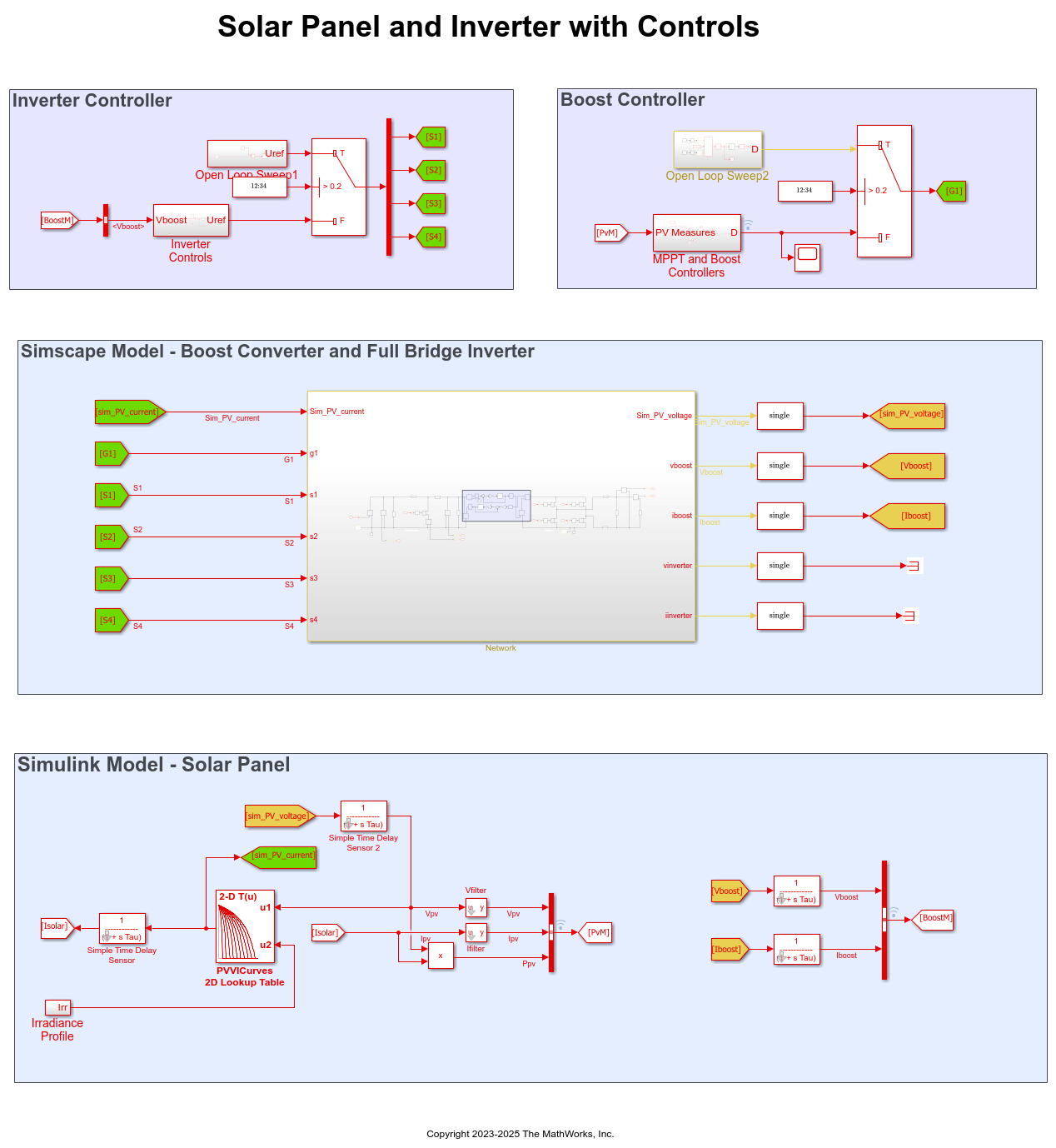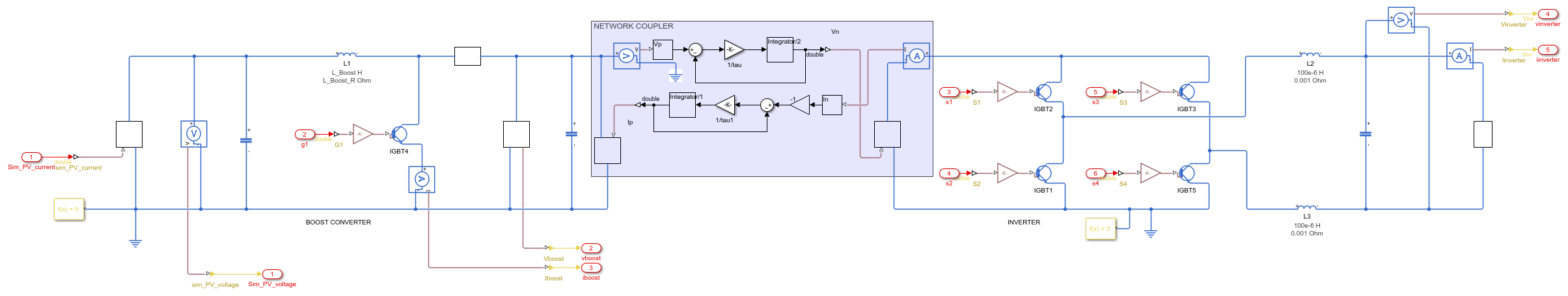# Generate HDL Code for Simscape Models with Multiple Networks

This example shows how you can run the Simscape HDL Workflow Advisor to generate the HDL implementation model for a Simscape™ model that contains multiple networks. You can also generate a validation logic that numerically compares each Simscape network with the corresponding state-space implementation in the HDL implementation model. The Simscape model in this example is a solar power inverter partitioned into two networks. To learn how this network is partitioned, see Partition Simscape Models Containing a Large Network into Multiple Smaller Networks.

### Why Use a Simscape Model with Multiple Networks

When your Simscape model contains many switching elements, the state-space representation can contain a large number of modes. The generated HDL implementation model for such a large design can consume a significantly large number of resources, and may even fail to synthesize on the target FPGA device. To reduce the number of modes, you can partition the Simscape network in your model into multiple networks, and then run the Simscape HDL Workflow Advisor.

### Solar Power Inverter Model with Multiple Networks

To open the model that contains multiple networks, run:

```open_system('sschdlexSolarInverterPartitionedNetworkExample') ```

For this example, the model is saved as Solar_Power_Inverter_Multiple_Network_HDL. This model is the same as sschdlexSolarInverterPartitionedNetworkExample but has the subsystems rearranged and the logic for the solar panel placed inside a Solar_Panel subsystem.

```open_system('Solar_Power_Inverter_Multiple_Network_HDL') set_param('Solar_Power_Inverter_Multiple_Network_HDL', 'SimulationCommand', 'Update') ```The model consists of four parts: solar panel, boost controller, inverter controller, and a boost converter and full bridge inverter. The solar panel is modeled in Simulink® by using lookup tables. The boost controller and inverter controller provide the control signals for the boost converter and the full bridge inverter which is an H-bridge.

The original model contains the boost converter and full bridge inverter as a single network inside one subsystem. To see this model, enter:

```open_system('sschdlexSolarInverterSingleNetworkExample') ```

The partitioned model contains the two networks inside separate subsystems. To see the boost converter, open the PV Boost subsystem.

```open_system('Solar_Power_Inverter_Multiple_Network_HDL/PV Boost') ```To see the full bridge inverter, open the PV Inverter subsystem.

```open_system('Solar_Power_Inverter_Multiple_Network_HDL/PV Inverter') ```### Run Simscape HDL Workflow Advisor for Model with Multiple Networks

1. To open the Simscape HDL Workflow Advisor for the model, enter:

```sschdladvisor('Solar_Power_Inverter_Multiple_Network_HDL') ```
```### Running Simscape HDL Workflow Advisor for <a href="matlab:(Solar_Power_Inverter_Multiple_Network_HDL)">Solar_Power_Inverter_Multiple_Network_HDL</a> Updating Model Advisor cache... Model Advisor cache updated. For new customizations, to update the cache, use the Advisor.Manager.refresh_customizations method. ```

2. Run the workflow to the Check switched linear task.

The Simscape HDL Workflow Advisor lists the number of networks present in the model and the number of algebraic and differential variables for each network. The Advisor uses the Solver Configuration block to identify each unique network in your model.3. Run the Extract equations task.

The task displays the number of modes, states, inputs, outputs, and differential variables for each Simscape network.4. Run the Discretize equations task.

The state-space representation now uses fewer modes. The number of modes is `58` for the boost converter and `9` for the full bridge inverter, which results in a total number of `67` modes. The reduction in the number of modes saves area of the HDL implementation model on the target device.5. Change the Validation logic tolerance to `1e-4` and select the Generate validation logic for the implementation model check box. Run the Generate implementation model task.### Open HDL Implementation Model and Validate HDL Algorithm

To open the implementation model, click the link in the Generate implementation model task log. Rename the model as Solar_Power_Inverter_Multiple_Network_StateSpace.

```open_system('Solar_Power_Inverter_Multiple_Network_StateSpace') set_param('Solar_Power_Inverter_Multiple_Network_StateSpace', 'SimulationCommand', 'Update') ```The model contains two HDL Subsystems. The HDL Subsystem block models the state-space equations for the boost converter. The HDL Subsystem1 block models the state-space equations for the full bridge inverter. The Validation and Validation1 subsystems compare functional equivalence of the state space representation of the boost converter and full bridge inverter with the corresponding Simscape network in the original model.

The state-space parameters are saved in a MAT file Solar_multiple_network_stateSpaceParameters.mat. The file contains a cell array of two structures. One structure contains the parameters for the boost converter. The other structure contains the parameters for the full bridge inverter.

To compare the functional equivalence, simulate the model. If simulating the model produces assertions, you can resolve the validation mismatch by modifying a combination of various settings in the Generate implementation model task until the HDL implementation model matches the Simscape algorithm. The settings include increasing the validation logic tolerance, increasing the number of solver iterations, and changing the floating-point precision. For more information, see Validate HDL Implementation Model to Simscape Algorithm.

### Generate HDL Code and Validation Model

1. Enable generation of the resource utilization report.

```hdlset_param('Solar_Power_Inverter_Multiple_Network_StateSpace', 'ResourceReport', 'on') ```

2. Before you generate HDL code, it is recommended that you enable generation of the validation model. The validation model compares the output of the generated model after code generation to the output of the original model. To learn more, see Generated Model and Validation Model.

```HDLmodelname = 'Solar_Power_Inverter_Multiple_Network_StateSpace'; hdlset_param(HDLmodelname, 'TargetDirectory', 'C:/Temp/hdlsrc'); hdlset_param(HDLmodelname, 'GenerateValidationModel', 'on'); ```

3. Run the `makehdl` function to generate code. To generate HDL code for both HDL Subsystem blocks, you can place the blocks inside another top level subsystem and then generate HDL code. Name this subsystem as HDL_DUT.

```makehdl('Solar_Power_Inverter_Single_Network_StateSpace/HDL_DUT') ```

The generated HDL code and validation model are saved in C:/Temp/hdlsrc directory. The generated code is saved as HDL_DUT_tc.vhd. To open the validation model, click the link to gm_Solar_Power_Inverter_Multiple_Network_StateSpace_vnl.slx in the code generation logs in the Command Window.

4. As you generate HDL code, open the Code Generation Report. The resource utilization report indicates a large amount of adders, multipliers, and registers that might be consumed on the target FPGA device.The overall resource consumption of the two networks is significantly less than the resource consumption of a single, large network. To learn about the resource consumption of the single solar power inverter network, see Partition Simscape Models Containing a Large Network into Multiple Smaller Networks.